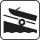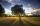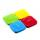MO - triangles

On the AB and AC sides of the triangle ABC lies successive points E and F, on segment EF lie point D. The EF and BC lines are parallel and is true this ratio FD:DE = AE:EB = 2:1. The area of ABC triangle is 27 hectares and line segments EF, AD, and DB segments are divided into four parts. Find the areas of these four parts.

Result

AED =  4 ha
BDE =  2 ha
BCFD =  13 ha

Solution:Leave us a comment of example and its solution (i.e. if it is still somewhat unclear...):Be the first to comment!Next similar examples:

1. Reverse Pythagorean theoremGiven are lengths of the sides of the triangles. Decide which one is rectangular: Δ ABC: 77 dm, 85 dm, 36 dm ? Δ DEF: 55 dm, 82 dm, 61 dm ? Δ GHI: 24 mm, 25 mm, 7 mm ? Δ JKL: 32 dm, 51 dm, 82 dm ? Δ MNO: 51 dm, 45 dm, 24 dm ?
2. Euclid theoremsCalculate the sides of a right triangle if leg a = 6 cm and a section of the hypotenuse, which is located adjacent the second leg b is 5cm.
3. RailwaysRailways climb 7.4 ‰. Calculate the height difference between two points on the railway distant 3539 meters.
4. Triangle KLBIt is given equilateral triangle ABC. From point L which is the midpoint of the side BC of the triangle it is drwn perpendicular to the side AB. Intersection of perpendicular and the side AB is point K. How many % of the area of the triangle ABC is area o
5. SimilarityAre two right triangles similar to each other if the first one has a acute angle 70° and second one has acute angle 20°?
6. HexagonThere is regular hexagon ABCDEF. If area of the triangle ABC is 22, what is area of the hexagon ABCDEF? I do not know how to solve it simply....
7. ClimbOn the road sign, which informs the climb is 8.7%. Car goes 5 km along this road. What is the height difference that car went?
8. Similarity coefficientThe ratio of similarity of two equilateral triangles is 3.5 (ie 7:2). The length of the side of smaller triangle is 2.4 cm. Calculate the perimeter and area of ​​the larger triangle.
9. ClimbRoad has climbing 1:27. How big is a angle corresponds to this climbing?
10. GeodesistTriangle shaped field (triangle ABC) has side AB = 129 m. path XY is parallel to the side AB which divided triangle ABC into two parts with same area. What will be the length of the path XY? Help please geodesist ...
11. BoatA force of 300 kg (3000 N) is required to pull a boat up a ramp inclined at 14° with horizontal. How much does the boat weight?
12. Sun raysIf the sun's rays are at an angle 60° then famous Great Pyramid of Egypt (which is now high 137.3 meters) has 79.3 m long shadow. Calculate current height of neighboring chefren pyramid whose shadow is measured at the same time 78.8 m and the current hei
13. AirplaneAviator sees part of the earth's surface with an area of 200,000 square kilometers. How high he flies?
14. Trapezoid IVIn a trapezoid ABCD (AB||CD) is |AB| = 15cm |CD| = 7 cm, |AC| = 12 cm, AC is perpendicular to BC. What area has a trapezoid ABCD?Nine meters height poplar tree has a shadow 16.2 meters long. How long shadow have at the same time Joe if he is 1,4m tall?Circular cone of height 15 cm and volume 5699 cm3 is at one-third of the height (measured from the bottom) cut by a plane parallel to the base. Calculate the radius and circumference of the circular cut.The ratio of the similarity of the squares ABCD and KLMN is 2.5. Square KLMN area is greater than area of a square ABCD with side a: ?